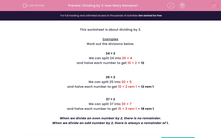# Dividing by 2: How Many Remains?

In this worksheet, students divide two-digit numbers by 2 and note if there are any remainders.This content is premium and exclusive to EdPlace subscribers.Key stage:  KS 2

Curriculum topic:   Maths and Numerical Reasoning

Curriculum subtopic:   Mixed Problems

Difficulty level:#### Worksheet Overview

This worksheet is about dividing by 2.

Examples

Work out the divisions below.

24 ÷ 2

We can split 24 into 20 + 4

and halve each number to get 10 + 2 = 12

25 ÷ 2

We can split 25 into 20 + 5

and halve each number to get 10 + 2 rem 1 = 12 rem 1

37 ÷ 2

We can split 37 into 30 + 7

and halve each number to get 15 + 3 rem 1 = 18 rem 1

When we divide an even number by 2, there is no remainder.

When we divide an odd number by 2, there is always a remainder of 1.

### What is EdPlace?

We're your National Curriculum aligned online education content provider helping each child succeed in English, maths and science from year 1 to GCSE. With an EdPlace account you’ll be able to track and measure progress, helping each child achieve their best. We build confidence and attainment by personalising each child’s learning at a level that suits them.

Get startedTry an activity or get started for free